Скачать презентацию Metal Complexes We know Lewis acids are

69ed723a5eded68085e6c56918a88c76.ppt

• Количество слайдов: 53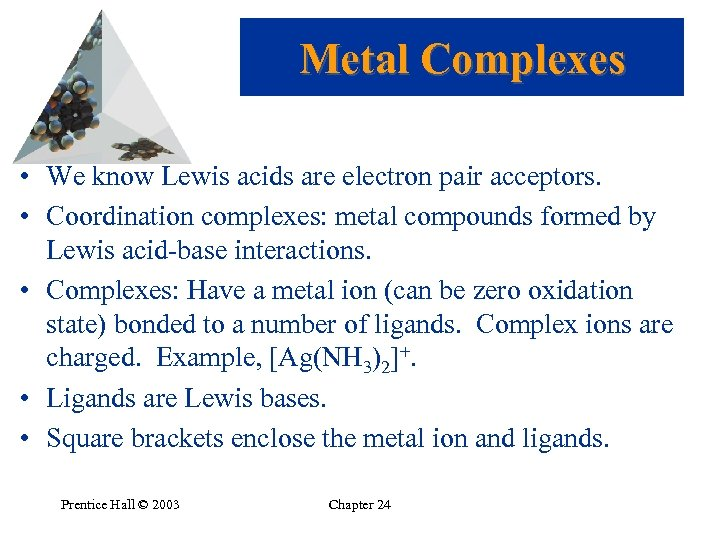Metal Complexes • We know Lewis acids are electron pair acceptors. • Coordination complexes: metal compounds formed by Lewis acid-base interactions. • Complexes: Have a metal ion (can be zero oxidation state) bonded to a number of ligands. Complex ions are charged. Example, [Ag(NH 3)2]+. • Ligands are Lewis bases. • Square brackets enclose the metal ion and ligands. Prentice Hall © 2003 Chapter 24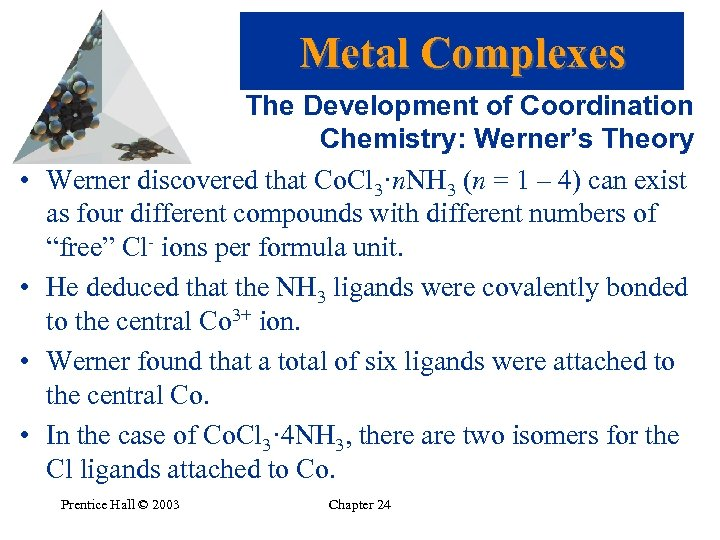Metal Complexes • • The Development of Coordination Chemistry: Werner’s Theory Werner discovered that Co. Cl 3·n. NH 3 (n = 1 – 4) can exist as four different compounds with different numbers of “free” Cl- ions per formula unit. He deduced that the NH 3 ligands were covalently bonded to the central Co 3+ ion. Werner found that a total of six ligands were attached to the central Co. In the case of Co. Cl 3· 4 NH 3, there are two isomers for the Cl ligands attached to Co. Prentice Hall © 2003 Chapter 24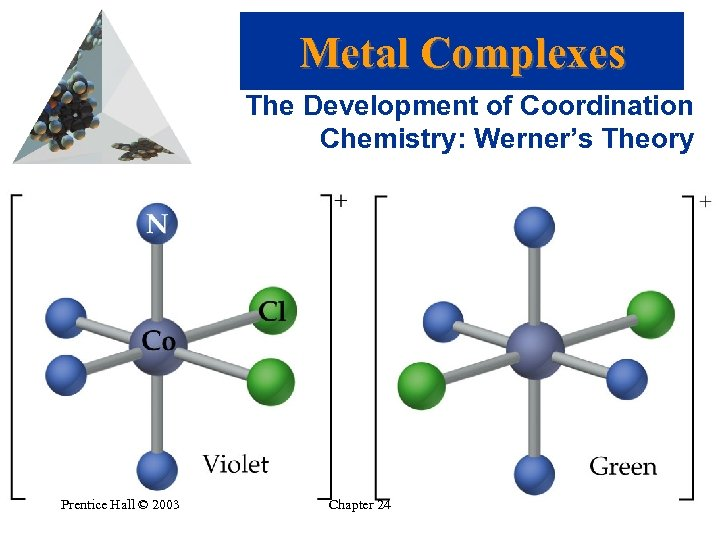Metal Complexes The Development of Coordination Chemistry: Werner’s Theory Prentice Hall © 2003 Chapter 24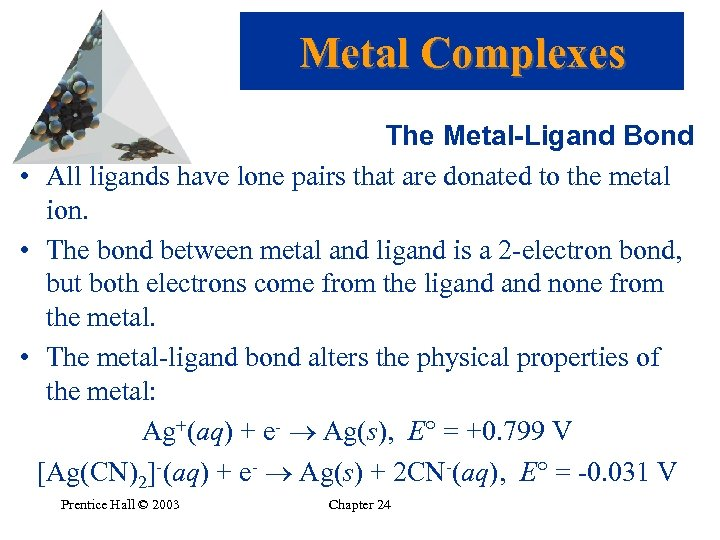Metal Complexes The Metal-Ligand Bond • All ligands have lone pairs that are donated to the metal ion. • The bond between metal and ligand is a 2 -electron bond, but both electrons come from the ligand none from the metal. • The metal-ligand bond alters the physical properties of the metal: Ag+(aq) + e- Ag(s), E = +0. 799 V [Ag(CN)2]-(aq) + e- Ag(s) + 2 CN-(aq), E = -0. 031 V Prentice Hall © 2003 Chapter 24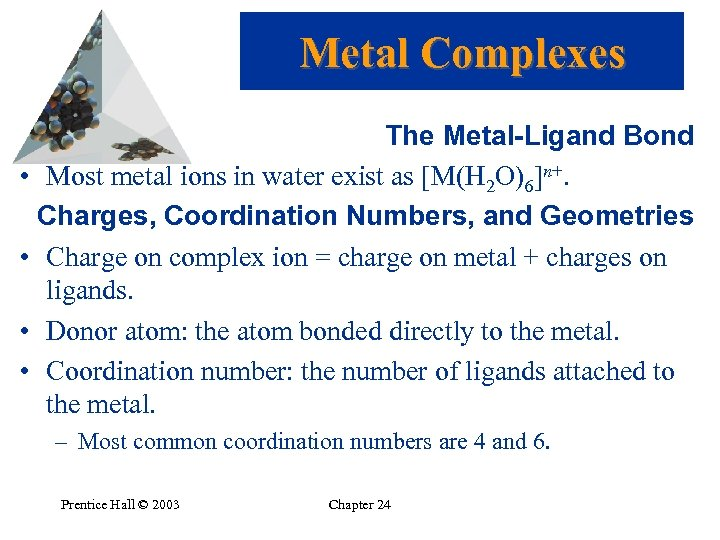Metal Complexes The Metal-Ligand Bond • Most metal ions in water exist as [M(H 2 O)6]n+. Charges, Coordination Numbers, and Geometries • Charge on complex ion = charge on metal + charges on ligands. • Donor atom: the atom bonded directly to the metal. • Coordination number: the number of ligands attached to the metal. – Most common coordination numbers are 4 and 6. Prentice Hall © 2003 Chapter 24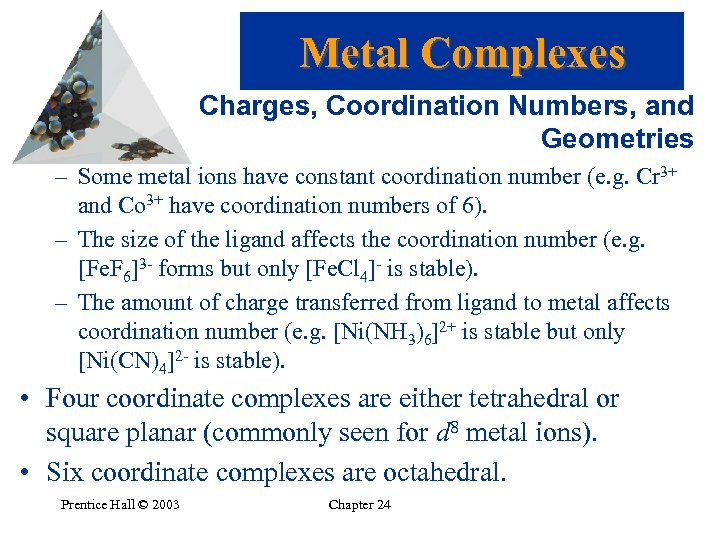Metal Complexes Charges, Coordination Numbers, and Geometries – Some metal ions have constant coordination number (e. g. Cr 3+ and Co 3+ have coordination numbers of 6). – The size of the ligand affects the coordination number (e. g. [Fe. F 6]3 - forms but only [Fe. Cl 4]- is stable). – The amount of charge transferred from ligand to metal affects coordination number (e. g. [Ni(NH 3)6]2+ is stable but only [Ni(CN)4]2 - is stable). • Four coordinate complexes are either tetrahedral or square planar (commonly seen for d 8 metal ions). • Six coordinate complexes are octahedral. Prentice Hall © 2003 Chapter 24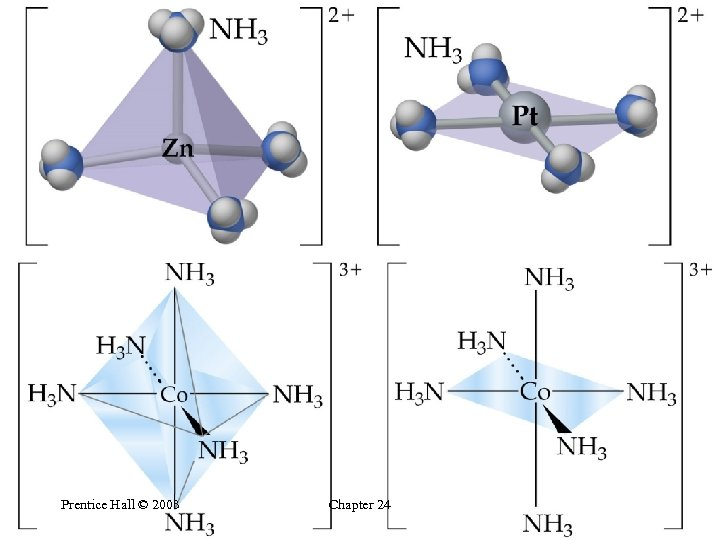Prentice Hall © 2003 Chapter 24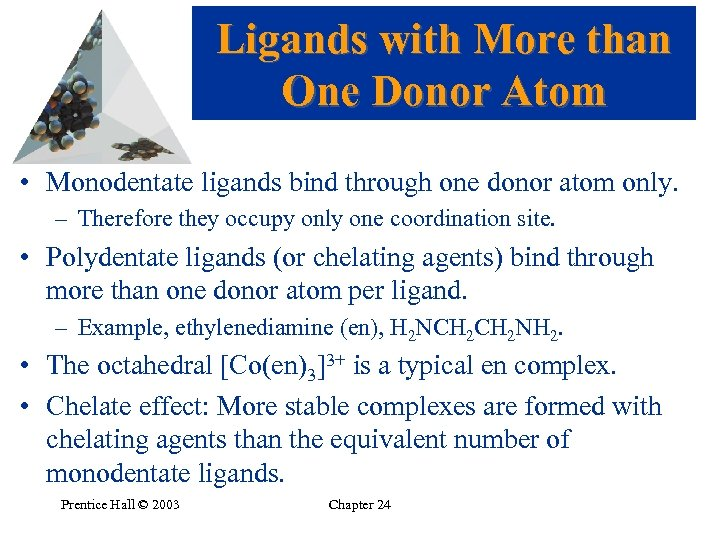Ligands with More than One Donor Atom • Monodentate ligands bind through one donor atom only. – Therefore they occupy only one coordination site. • Polydentate ligands (or chelating agents) bind through more than one donor atom per ligand. – Example, ethylenediamine (en), H 2 NCH 2 NH 2. • The octahedral [Co(en)3]3+ is a typical en complex. • Chelate effect: More stable complexes are formed with chelating agents than the equivalent number of monodentate ligands. Prentice Hall © 2003 Chapter 24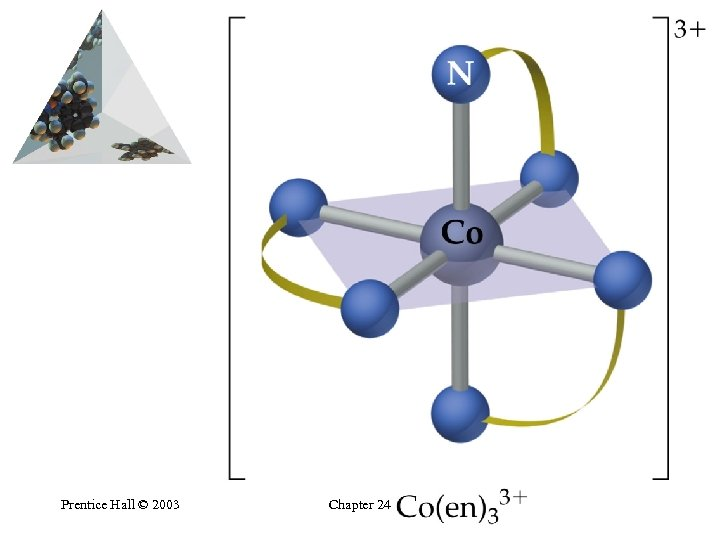Prentice Hall © 2003 Chapter 24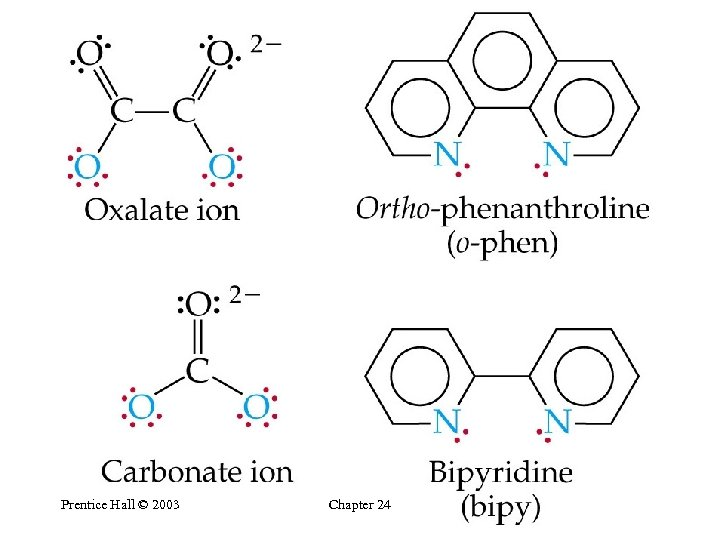Prentice Hall © 2003 Chapter 24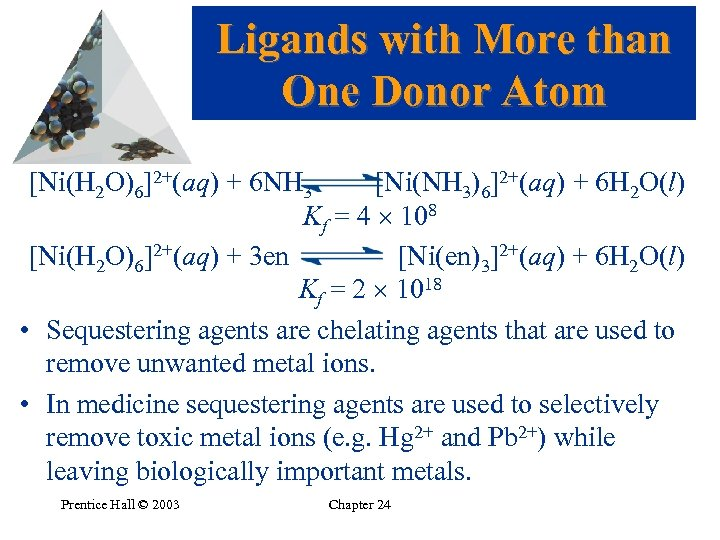Ligands with More than One Donor Atom [Ni(H 2 O)6]2+(aq) + 6 NH 3 [Ni(NH 3)6]2+(aq) + 6 H 2 O(l) Kf = 4 108 [Ni(H 2 O)6]2+(aq) + 3 en [Ni(en)3]2+(aq) + 6 H 2 O(l) Kf = 2 1018 • Sequestering agents are chelating agents that are used to remove unwanted metal ions. • In medicine sequestering agents are used to selectively remove toxic metal ions (e. g. Hg 2+ and Pb 2+) while leaving biologically important metals. Prentice Hall © 2003 Chapter 24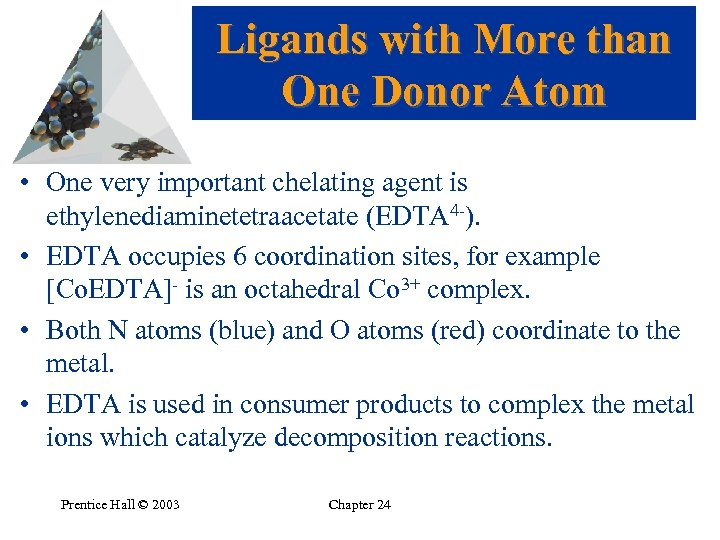Ligands with More than One Donor Atom • One very important chelating agent is ethylenediaminetetraacetate (EDTA 4 -). • EDTA occupies 6 coordination sites, for example [Co. EDTA]- is an octahedral Co 3+ complex. • Both N atoms (blue) and O atoms (red) coordinate to the metal. • EDTA is used in consumer products to complex the metal ions which catalyze decomposition reactions. Prentice Hall © 2003 Chapter 24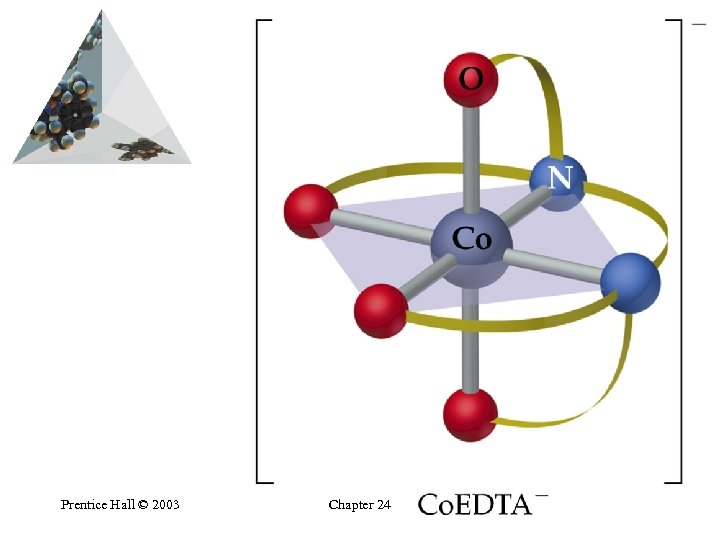Prentice Hall © 2003 Chapter 24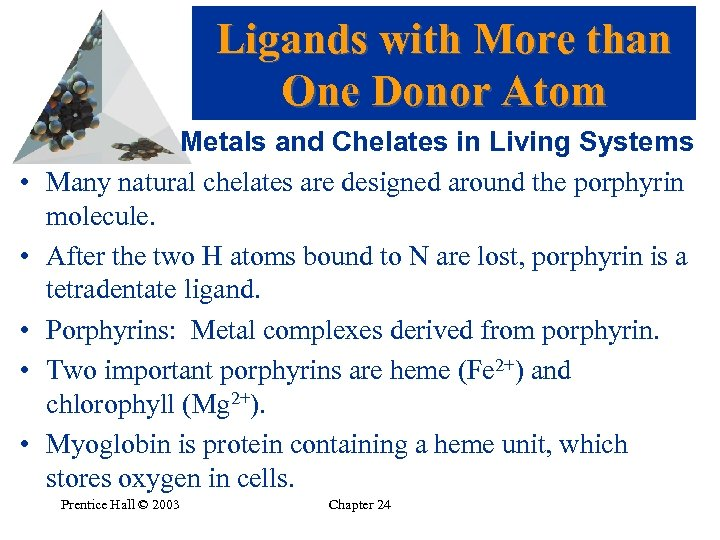Ligands with More than One Donor Atom • • • Metals and Chelates in Living Systems Many natural chelates are designed around the porphyrin molecule. After the two H atoms bound to N are lost, porphyrin is a tetradentate ligand. Porphyrins: Metal complexes derived from porphyrin. Two important porphyrins are heme (Fe 2+) and chlorophyll (Mg 2+). Myoglobin is protein containing a heme unit, which stores oxygen in cells. Prentice Hall © 2003 Chapter 24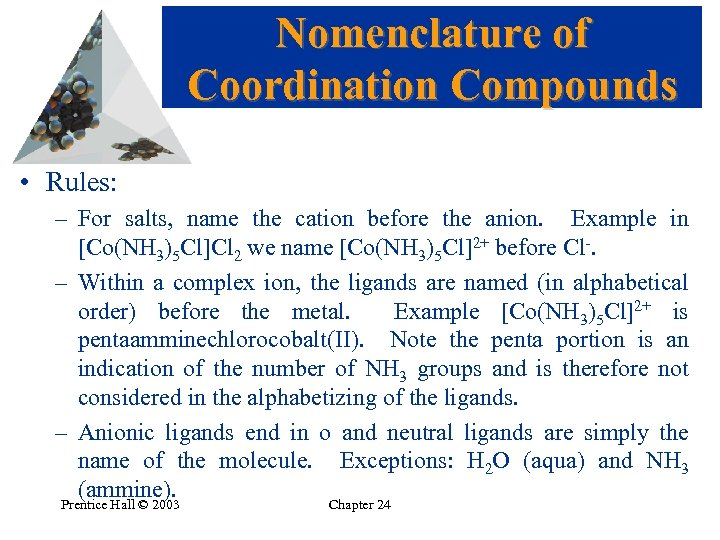Nomenclature of Coordination Compounds • Rules: – For salts, name the cation before the anion. Example in [Co(NH 3)5 Cl]Cl 2 we name [Co(NH 3)5 Cl]2+ before Cl-. – Within a complex ion, the ligands are named (in alphabetical order) before the metal. Example [Co(NH 3)5 Cl]2+ is pentaamminechlorocobalt(II). Note the penta portion is an indication of the number of NH 3 groups and is therefore not considered in the alphabetizing of the ligands. – Anionic ligands end in o and neutral ligands are simply the name of the molecule. Exceptions: H 2 O (aqua) and NH 3 (ammine). Prentice Hall © 2003 Chapter 24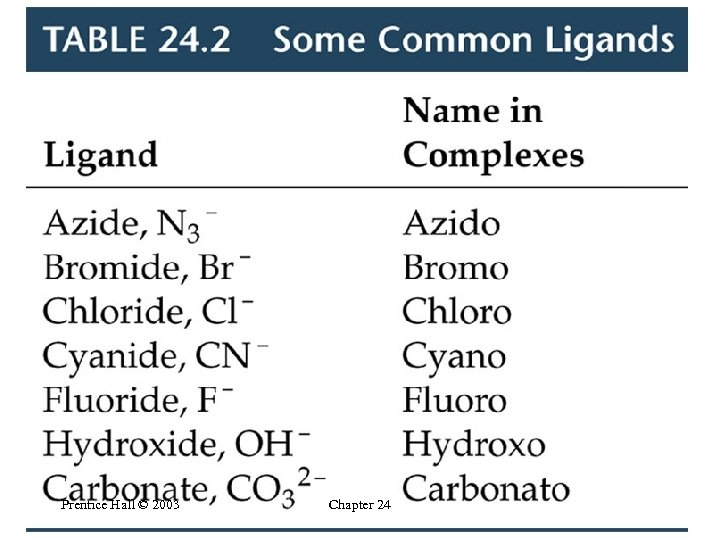Prentice Hall © 2003 Chapter 24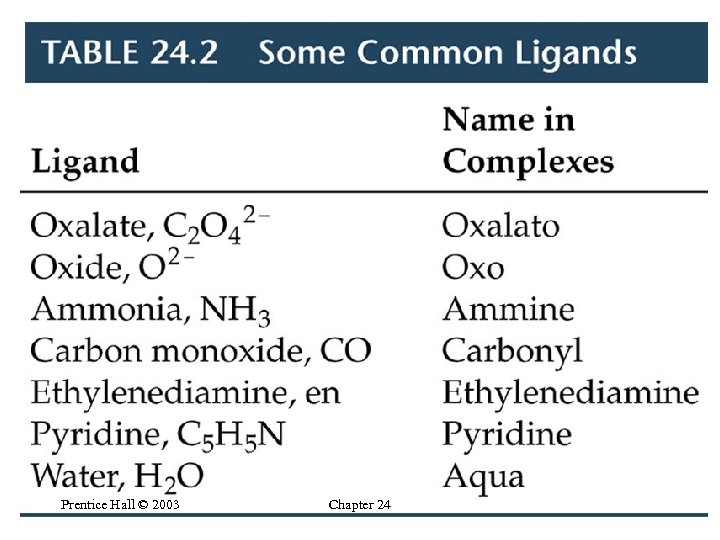Prentice Hall © 2003 Chapter 24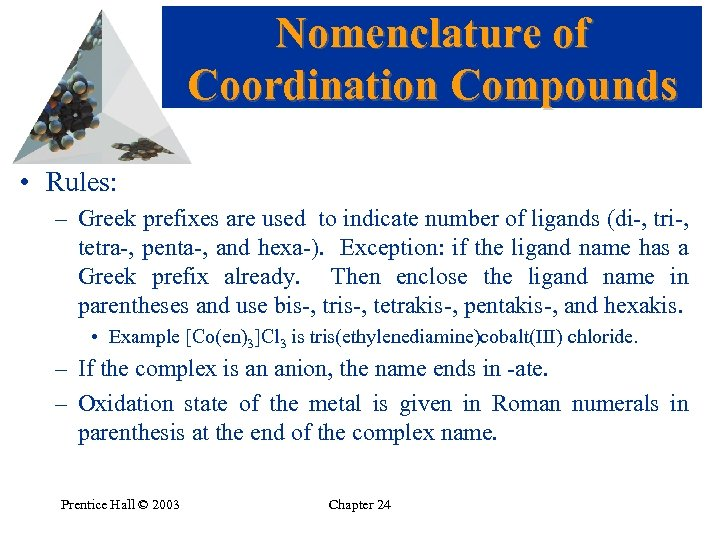Nomenclature of Coordination Compounds • Rules: – Greek prefixes are used to indicate number of ligands (di-, tri-, tetra-, penta-, and hexa-). Exception: if the ligand name has a Greek prefix already. Then enclose the ligand name in parentheses and use bis-, tris-, tetrakis-, pentakis-, and hexakis. • Example [Co(en)3]Cl 3 is tris(ethylenediamine)cobalt(III) chloride. – If the complex is an anion, the name ends in -ate. – Oxidation state of the metal is given in Roman numerals in parenthesis at the end of the complex name. Prentice Hall © 2003 Chapter 24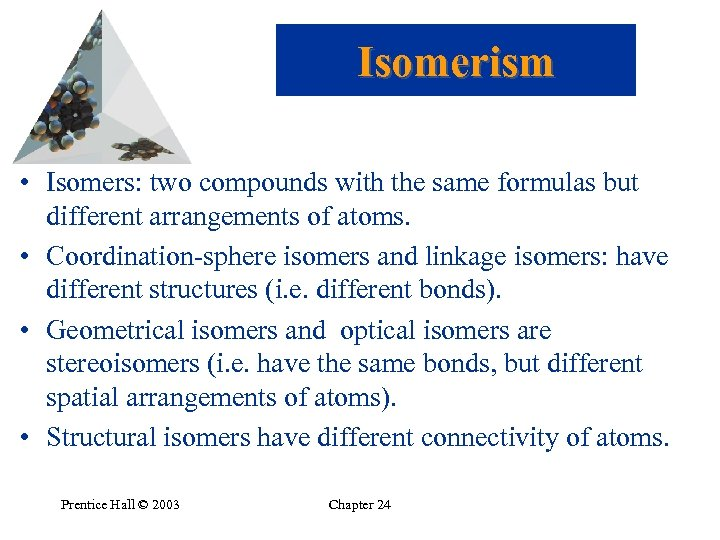Isomerism • Isomers: two compounds with the same formulas but different arrangements of atoms. • Coordination-sphere isomers and linkage isomers: have different structures (i. e. different bonds). • Geometrical isomers and optical isomers are stereoisomers (i. e. have the same bonds, but different spatial arrangements of atoms). • Structural isomers have different connectivity of atoms. Prentice Hall © 2003 Chapter 24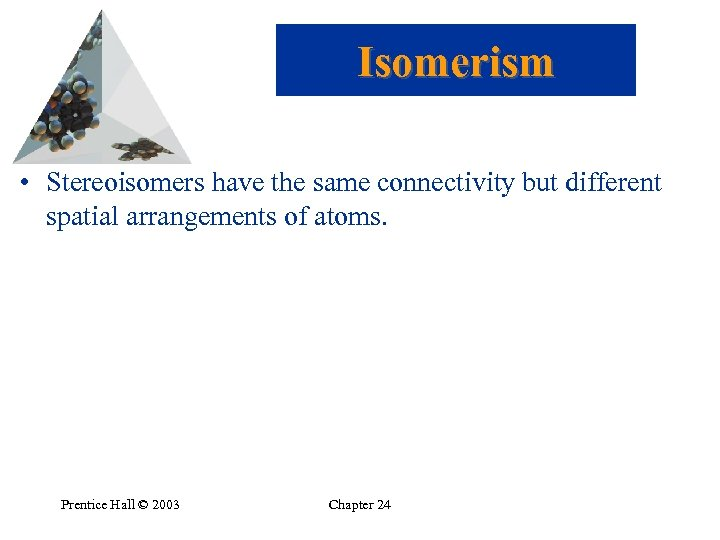Isomerism • Stereoisomers have the same connectivity but different spatial arrangements of atoms. Prentice Hall © 2003 Chapter 24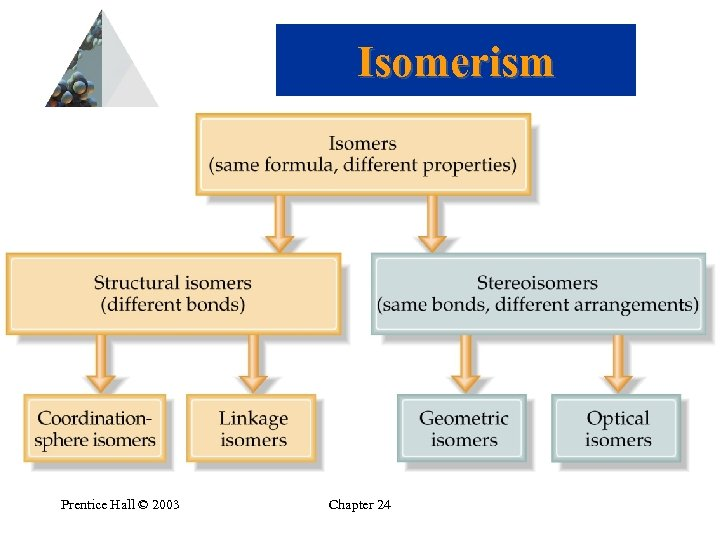Isomerism Prentice Hall © 2003 Chapter 24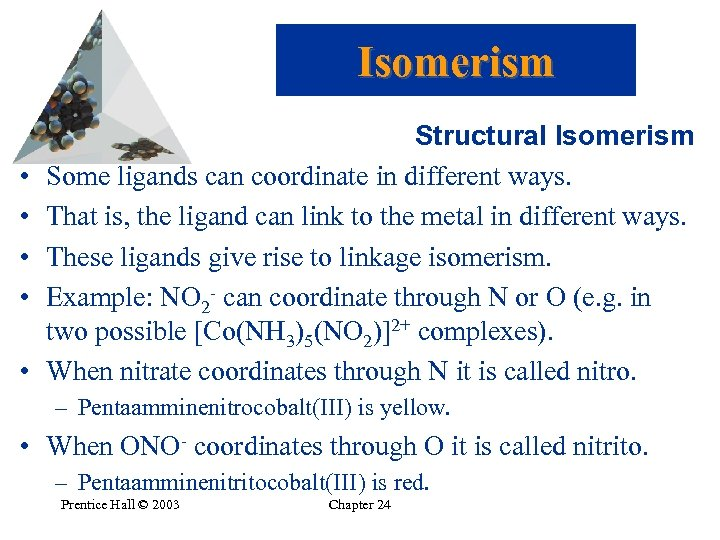Isomerism • • • Structural Isomerism Some ligands can coordinate in different ways. That is, the ligand can link to the metal in different ways. These ligands give rise to linkage isomerism. Example: NO 2 - can coordinate through N or O (e. g. in two possible [Co(NH 3)5(NO 2)]2+ complexes). When nitrate coordinates through N it is called nitro. – Pentaamminenitrocobalt(III) is yellow. • When ONO- coordinates through O it is called nitrito. – Pentaamminenitritocobalt(III) is red. Prentice Hall © 2003 Chapter 24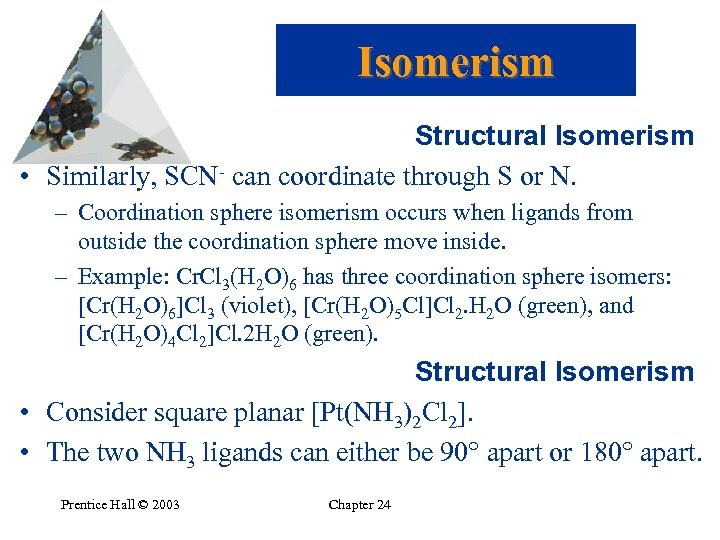Isomerism Structural Isomerism • Similarly, SCN- can coordinate through S or N. – Coordination sphere isomerism occurs when ligands from outside the coordination sphere move inside. – Example: Cr. Cl 3(H 2 O)6 has three coordination sphere isomers: [Cr(H 2 O)6]Cl 3 (violet), [Cr(H 2 O)5 Cl]Cl 2. H 2 O (green), and [Cr(H 2 O)4 Cl 2]Cl. 2 H 2 O (green). Structural Isomerism • Consider square planar [Pt(NH 3)2 Cl 2]. • The two NH 3 ligands can either be 90 apart or 180 apart. Prentice Hall © 2003 Chapter 24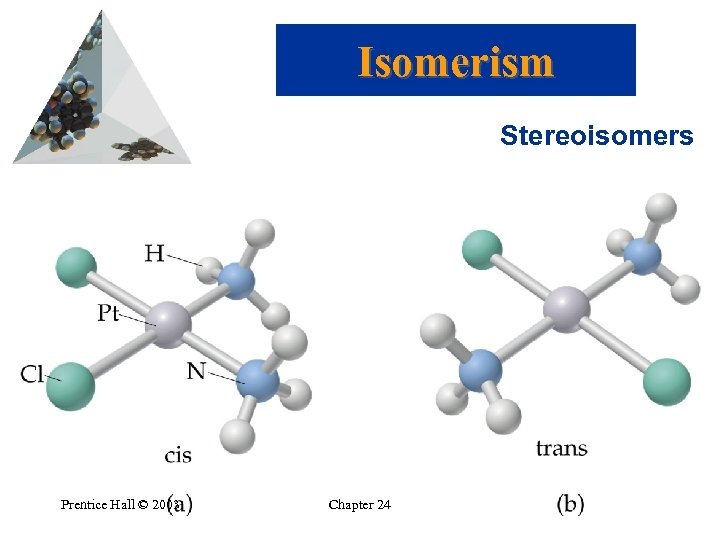Isomerism Stereoisomers Prentice Hall © 2003 Chapter 24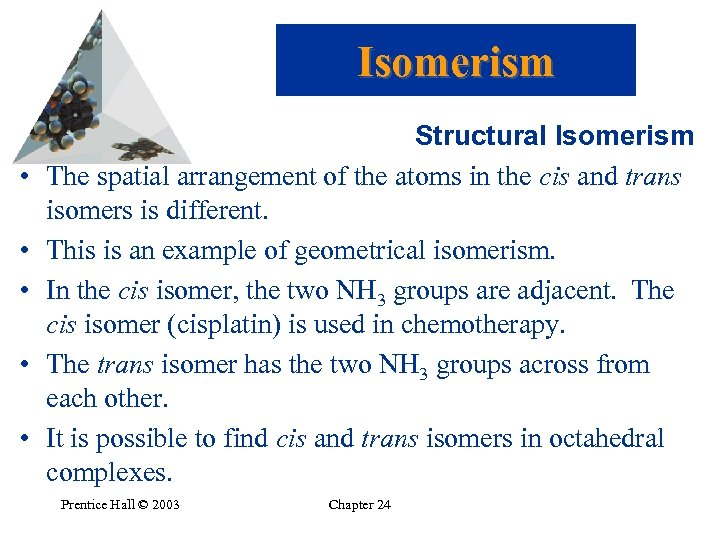Isomerism • • • Structural Isomerism The spatial arrangement of the atoms in the cis and trans isomers is different. This is an example of geometrical isomerism. In the cis isomer, the two NH 3 groups are adjacent. The cis isomer (cisplatin) is used in chemotherapy. The trans isomer has the two NH 3 groups across from each other. It is possible to find cis and trans isomers in octahedral complexes. Prentice Hall © 2003 Chapter 24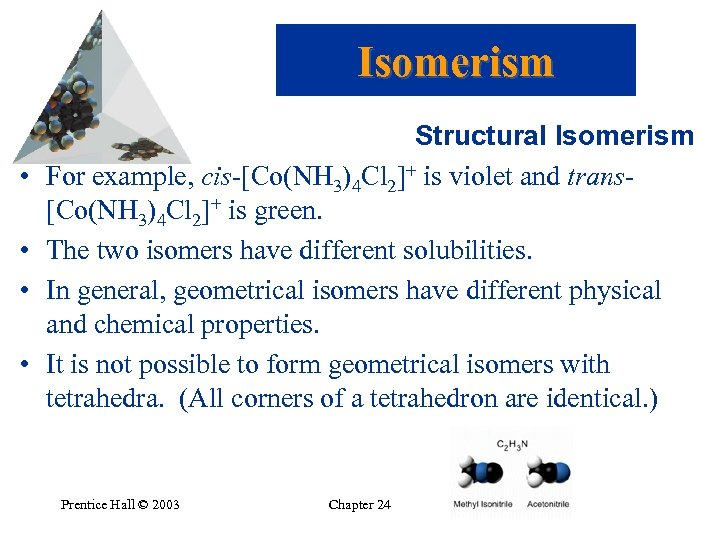Isomerism • • Structural Isomerism For example, cis-[Co(NH 3)4 Cl 2]+ is violet and trans[Co(NH 3)4 Cl 2]+ is green. The two isomers have different solubilities. In general, geometrical isomers have different physical and chemical properties. It is not possible to form geometrical isomers with tetrahedra. (All corners of a tetrahedron are identical. ) Prentice Hall © 2003 Chapter 24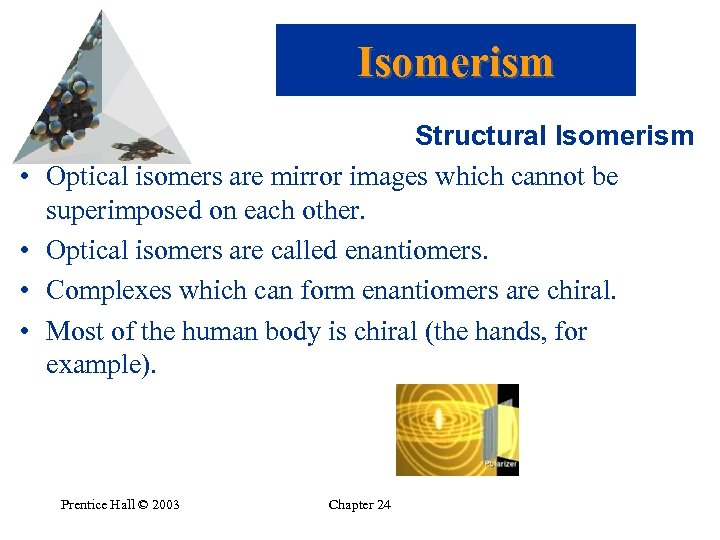Isomerism • • Structural Isomerism Optical isomers are mirror images which cannot be superimposed on each other. Optical isomers are called enantiomers. Complexes which can form enantiomers are chiral. Most of the human body is chiral (the hands, for example). Prentice Hall © 2003 Chapter 24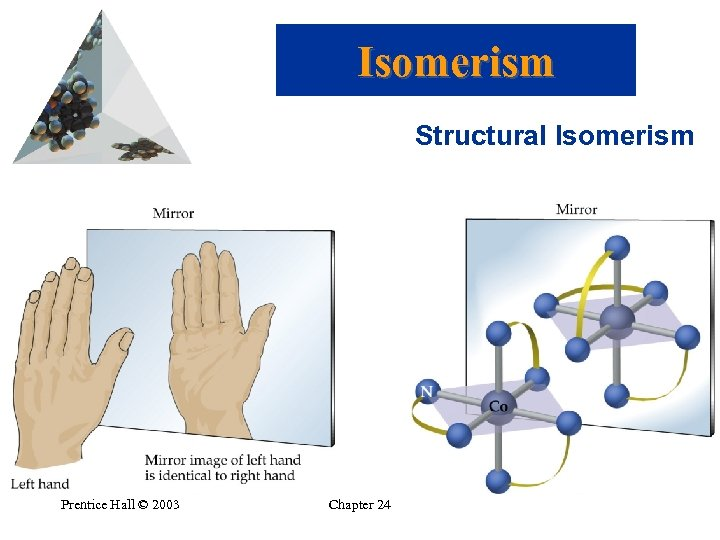Isomerism Structural Isomerism Prentice Hall © 2003 Chapter 24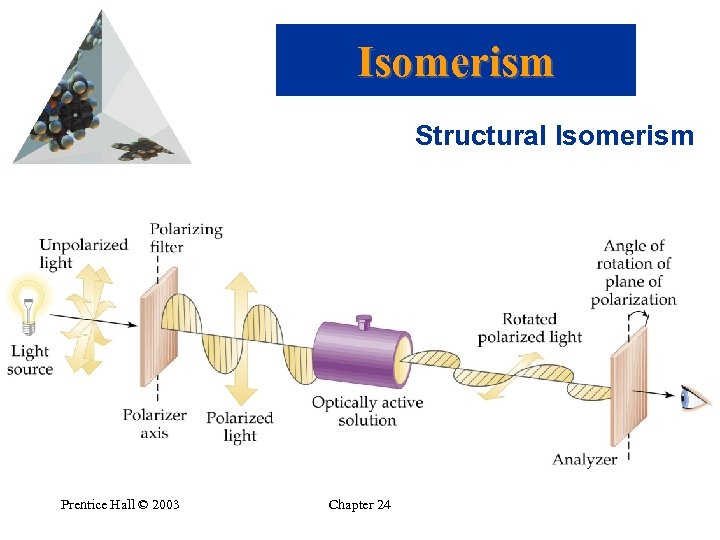Isomerism Structural Isomerism Prentice Hall © 2003 Chapter 24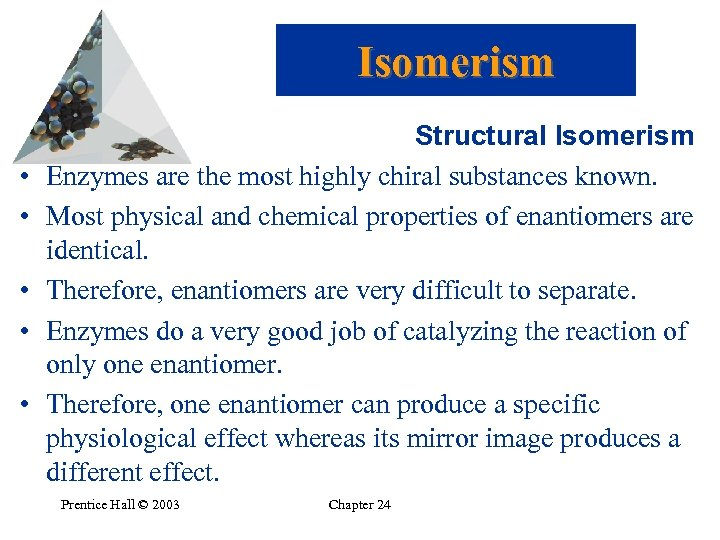Isomerism • • • Structural Isomerism Enzymes are the most highly chiral substances known. Most physical and chemical properties of enantiomers are identical. Therefore, enantiomers are very difficult to separate. Enzymes do a very good job of catalyzing the reaction of only one enantiomer. Therefore, one enantiomer can produce a specific physiological effect whereas its mirror image produces a different effect. Prentice Hall © 2003 Chapter 24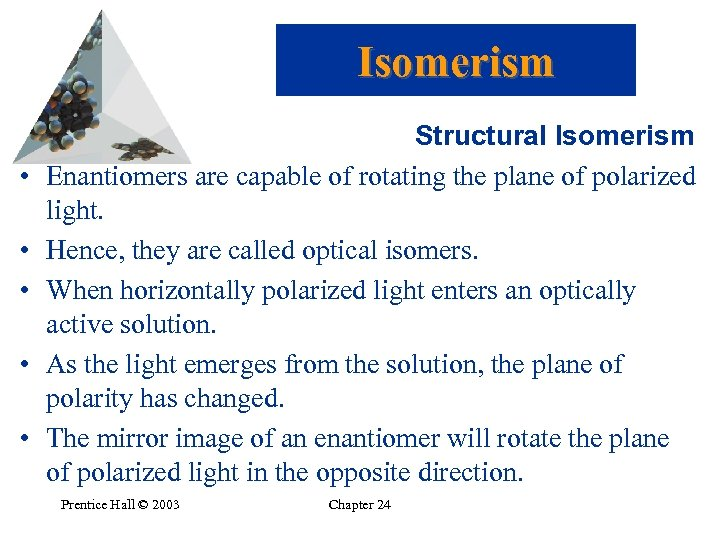Isomerism • • • Structural Isomerism Enantiomers are capable of rotating the plane of polarized light. Hence, they are called optical isomers. When horizontally polarized light enters an optically active solution. As the light emerges from the solution, the plane of polarity has changed. The mirror image of an enantiomer will rotate the plane of polarized light in the opposite direction. Prentice Hall © 2003 Chapter 24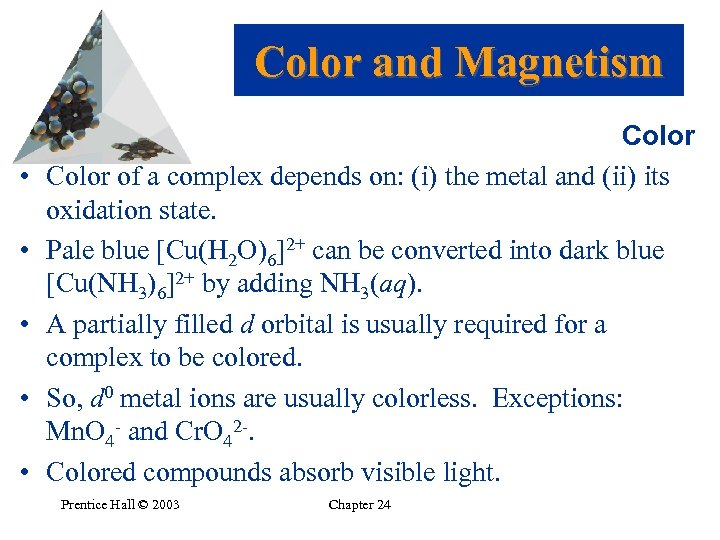Color and Magnetism • • • Color of a complex depends on: (i) the metal and (ii) its oxidation state. Pale blue [Cu(H 2 O)6]2+ can be converted into dark blue [Cu(NH 3)6]2+ by adding NH 3(aq). A partially filled d orbital is usually required for a complex to be colored. So, d 0 metal ions are usually colorless. Exceptions: Mn. O 4 - and Cr. O 42 -. Colored compounds absorb visible light. Prentice Hall © 2003 Chapter 24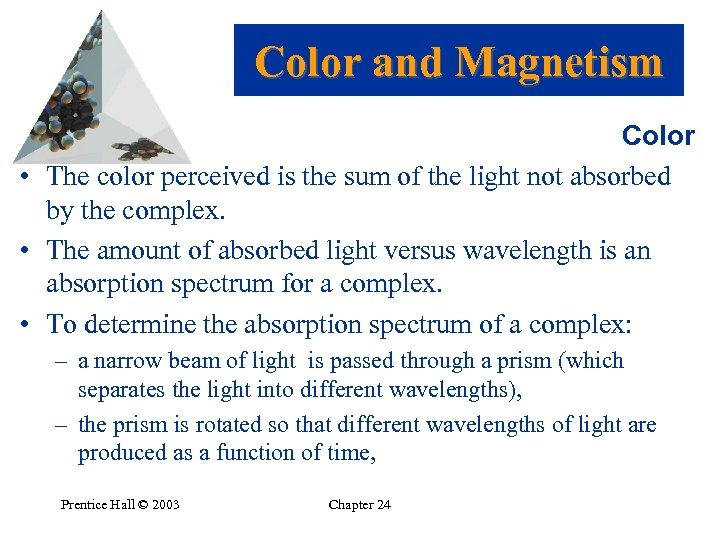Color and Magnetism Color • The color perceived is the sum of the light not absorbed by the complex. • The amount of absorbed light versus wavelength is an absorption spectrum for a complex. • To determine the absorption spectrum of a complex: – a narrow beam of light is passed through a prism (which separates the light into different wavelengths), – the prism is rotated so that different wavelengths of light are produced as a function of time, Prentice Hall © 2003 Chapter 24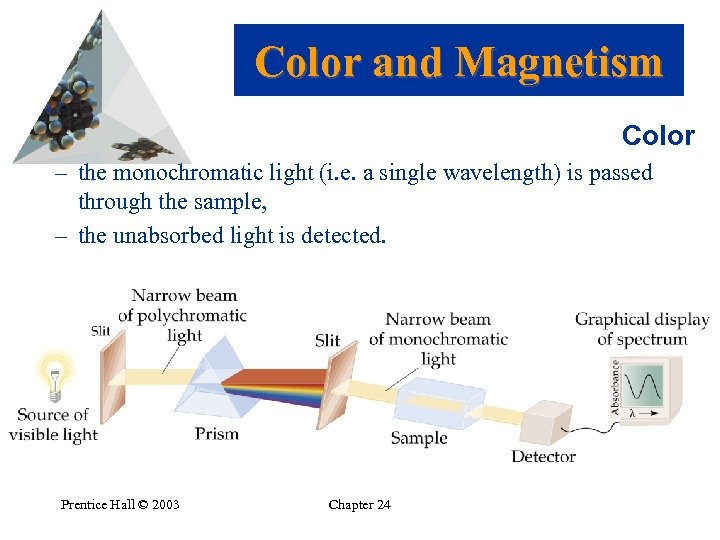Color and Magnetism Color – the monochromatic light (i. e. a single wavelength) is passed through the sample, – the unabsorbed light is detected. Prentice Hall © 2003 Chapter 24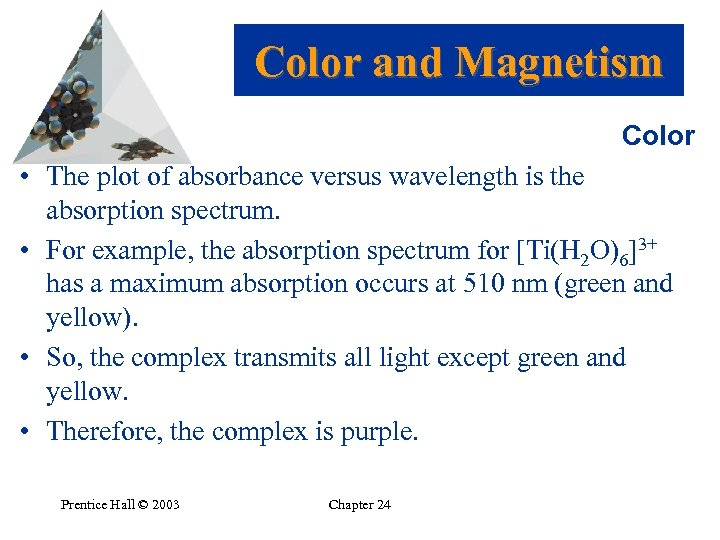Color and Magnetism Color • The plot of absorbance versus wavelength is the absorption spectrum. • For example, the absorption spectrum for [Ti(H 2 O)6]3+ has a maximum absorption occurs at 510 nm (green and yellow). • So, the complex transmits all light except green and yellow. • Therefore, the complex is purple. Prentice Hall © 2003 Chapter 24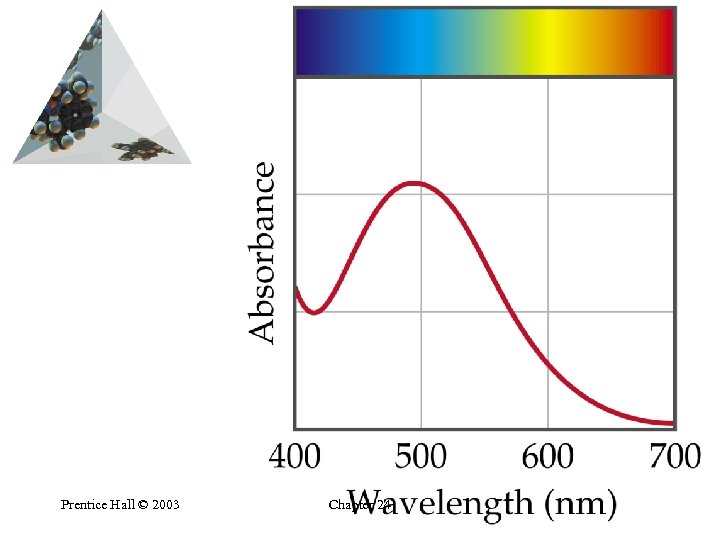Prentice Hall © 2003 Chapter 24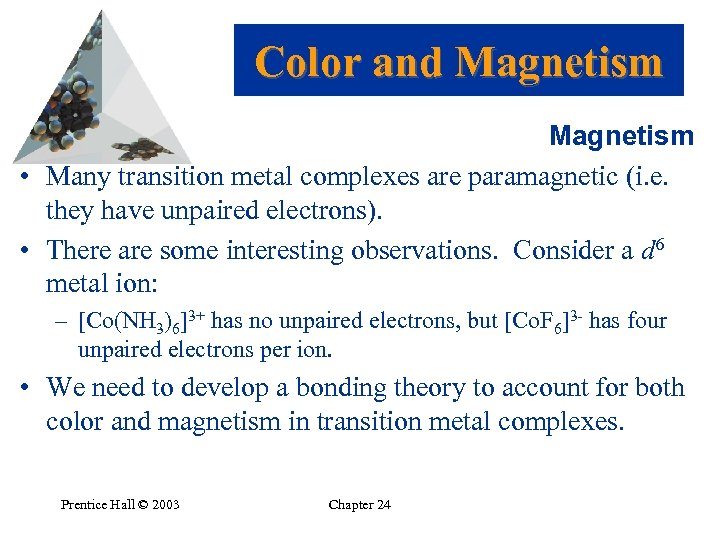Color and Magnetism • Many transition metal complexes are paramagnetic (i. e. they have unpaired electrons). • There are some interesting observations. Consider a d 6 metal ion: – [Co(NH 3)6]3+ has no unpaired electrons, but [Co. F 6]3 - has four unpaired electrons per ion. • We need to develop a bonding theory to account for both color and magnetism in transition metal complexes. Prentice Hall © 2003 Chapter 24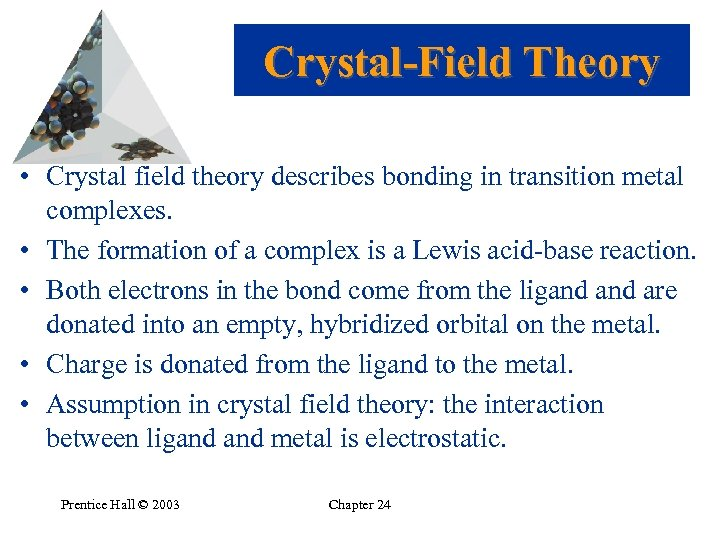Crystal-Field Theory • Crystal field theory describes bonding in transition metal complexes. • The formation of a complex is a Lewis acid-base reaction. • Both electrons in the bond come from the ligand are donated into an empty, hybridized orbital on the metal. • Charge is donated from the ligand to the metal. • Assumption in crystal field theory: the interaction between ligand metal is electrostatic. Prentice Hall © 2003 Chapter 24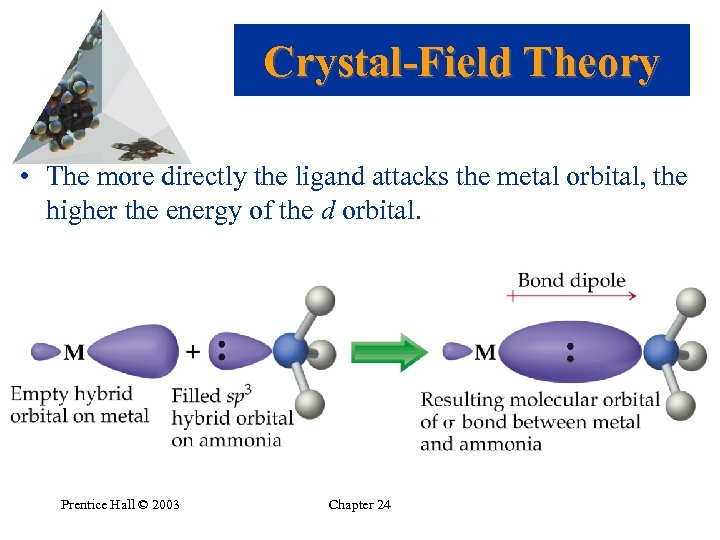Crystal-Field Theory • The more directly the ligand attacks the metal orbital, the higher the energy of the d orbital. Prentice Hall © 2003 Chapter 24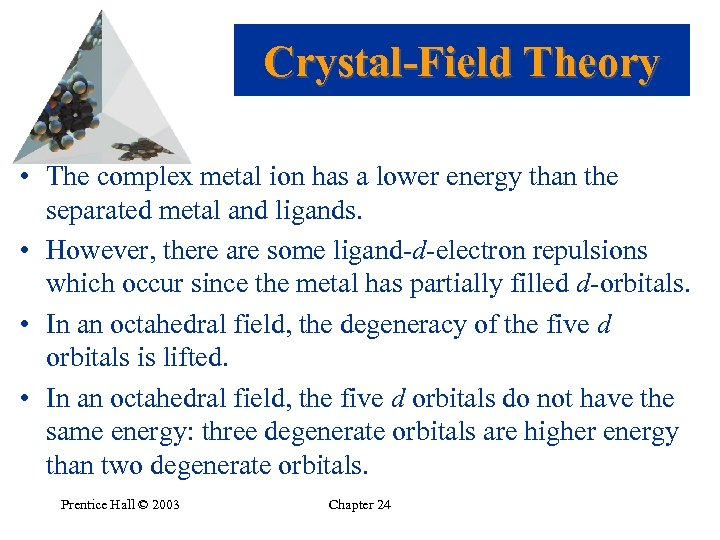Crystal-Field Theory • The complex metal ion has a lower energy than the separated metal and ligands. • However, there are some ligand-d-electron repulsions which occur since the metal has partially filled d-orbitals. • In an octahedral field, the degeneracy of the five d orbitals is lifted. • In an octahedral field, the five d orbitals do not have the same energy: three degenerate orbitals are higher energy than two degenerate orbitals. Prentice Hall © 2003 Chapter 24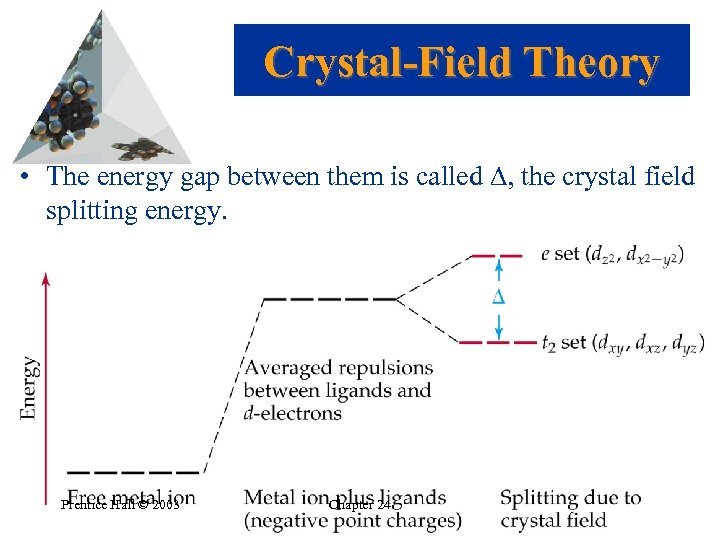Crystal-Field Theory • The energy gap between them is called , the crystal field splitting energy. Prentice Hall © 2003 Chapter 24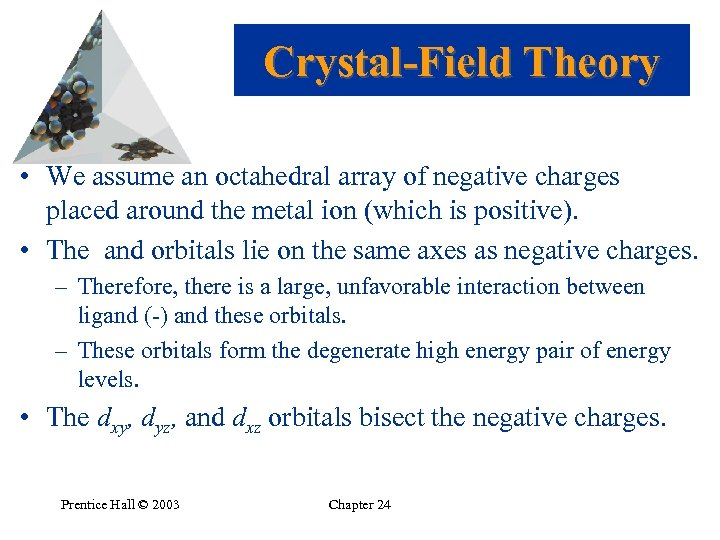Crystal-Field Theory • We assume an octahedral array of negative charges placed around the metal ion (which is positive). • The and orbitals lie on the same axes as negative charges. – Therefore, there is a large, unfavorable interaction between ligand (-) and these orbitals. – These orbitals form the degenerate high energy pair of energy levels. • The dxy, dyz, and dxz orbitals bisect the negative charges. Prentice Hall © 2003 Chapter 24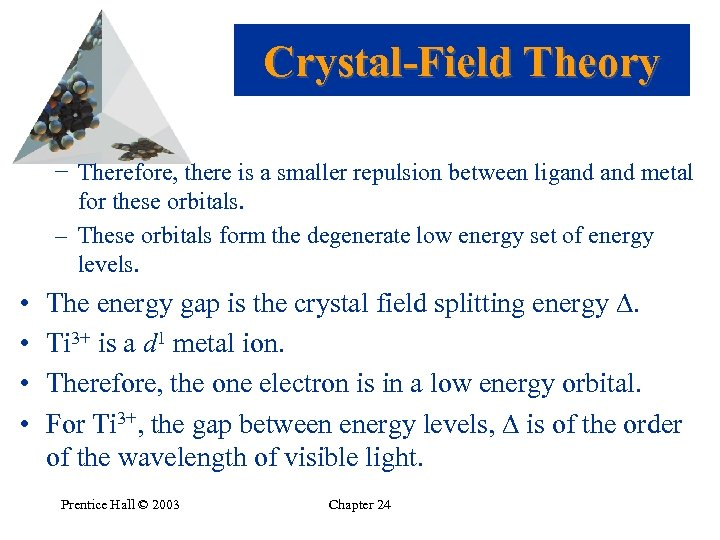Crystal-Field Theory − Therefore, there is a smaller repulsion between ligand metal for these orbitals. – These orbitals form the degenerate low energy set of energy levels. • • The energy gap is the crystal field splitting energy . Ti 3+ is a d 1 metal ion. Therefore, the one electron is in a low energy orbital. For Ti 3+, the gap between energy levels, is of the order of the wavelength of visible light. Prentice Hall © 2003 Chapter 24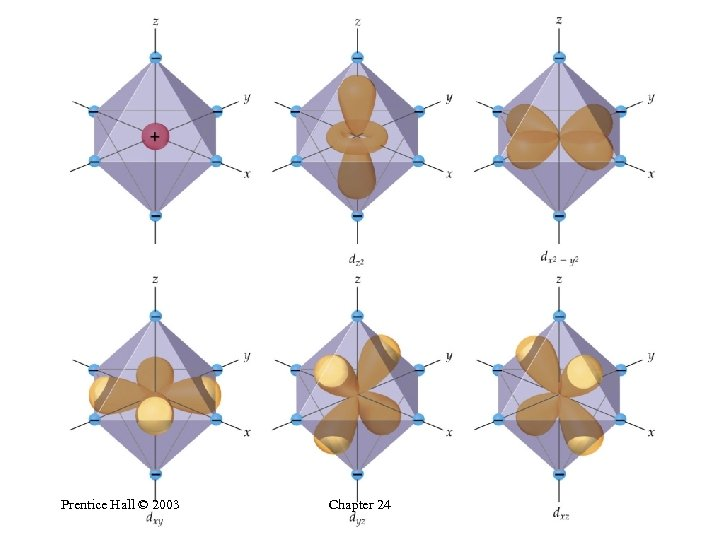Prentice Hall © 2003 Chapter 24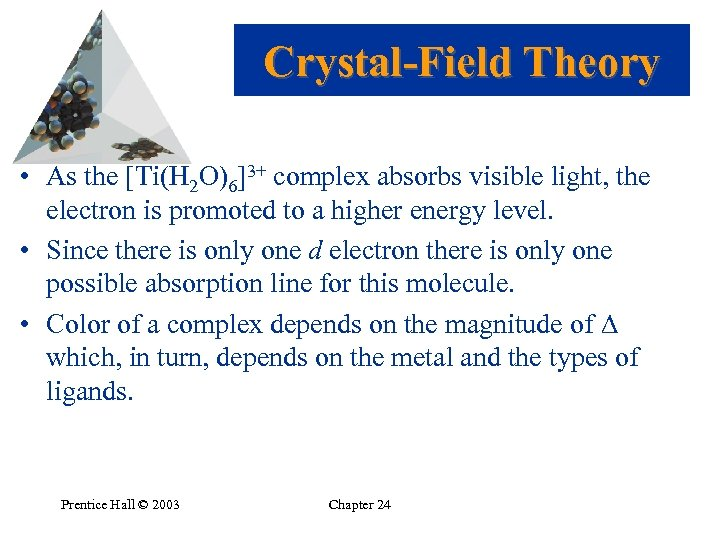Crystal-Field Theory • As the [Ti(H 2 O)6]3+ complex absorbs visible light, the electron is promoted to a higher energy level. • Since there is only one d electron there is only one possible absorption line for this molecule. • Color of a complex depends on the magnitude of which, in turn, depends on the metal and the types of ligands. Prentice Hall © 2003 Chapter 24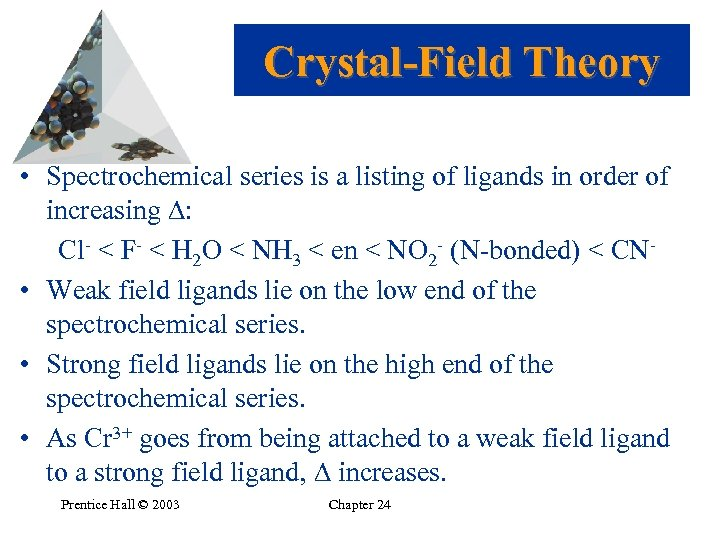Crystal-Field Theory • Spectrochemical series is a listing of ligands in order of increasing : Cl- < F- < H 2 O < NH 3 < en < NO 2 - (N-bonded) < CN • Weak field ligands lie on the low end of the spectrochemical series. • Strong field ligands lie on the high end of the spectrochemical series. • As Cr 3+ goes from being attached to a weak field ligand to a strong field ligand, increases. Prentice Hall © 2003 Chapter 24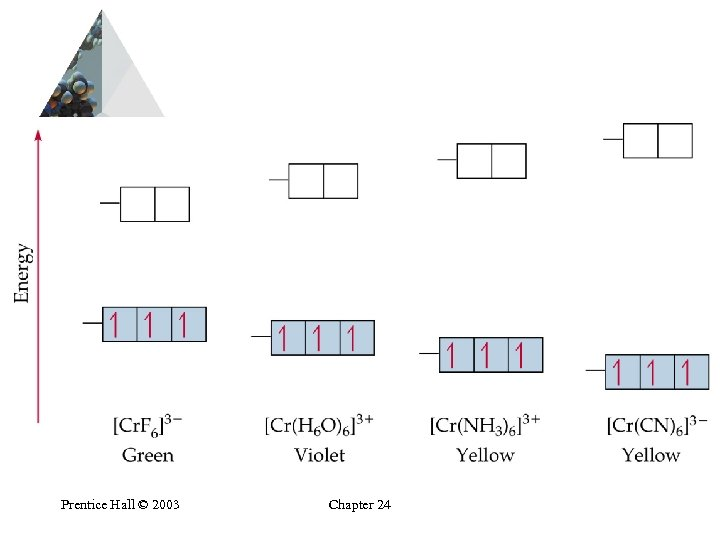Prentice Hall © 2003 Chapter 24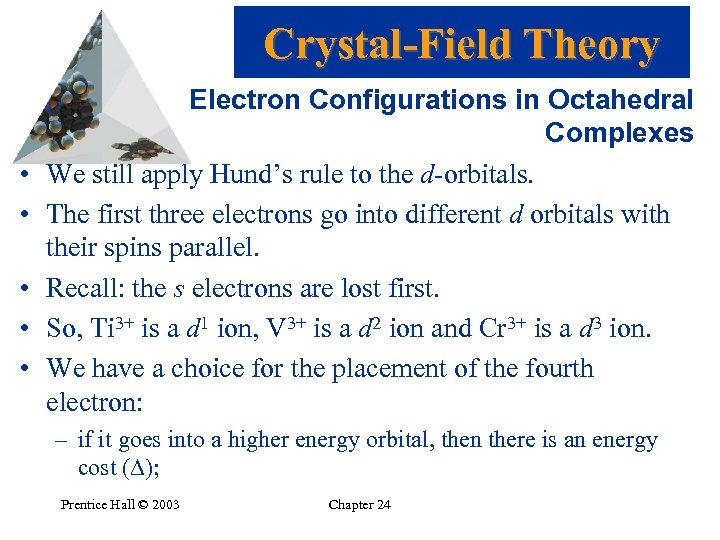Crystal-Field Theory • • • Electron Configurations in Octahedral Complexes We still apply Hund’s rule to the d-orbitals. The first three electrons go into different d orbitals with their spins parallel. Recall: the s electrons are lost first. So, Ti 3+ is a d 1 ion, V 3+ is a d 2 ion and Cr 3+ is a d 3 ion. We have a choice for the placement of the fourth electron: – if it goes into a higher energy orbital, then there is an energy cost ( ); Prentice Hall © 2003 Chapter 24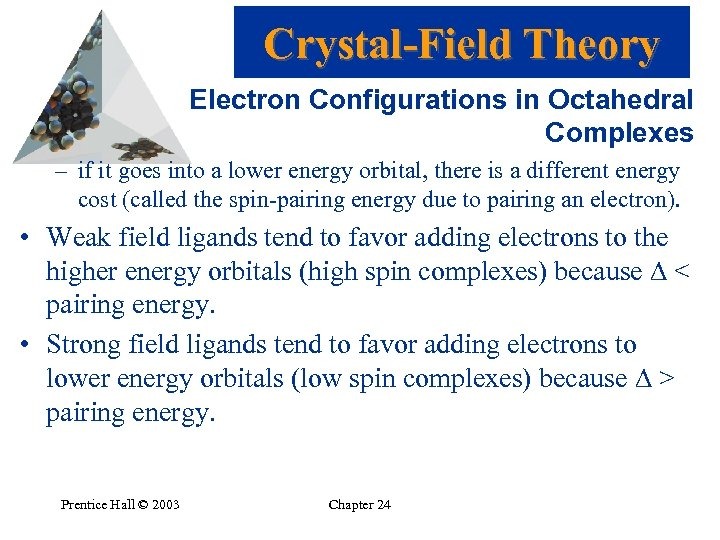Crystal-Field Theory Electron Configurations in Octahedral Complexes – if it goes into a lower energy orbital, there is a different energy cost (called the spin-pairing energy due to pairing an electron). • Weak field ligands tend to favor adding electrons to the higher energy orbitals (high spin complexes) because < pairing energy. • Strong field ligands tend to favor adding electrons to lower energy orbitals (low spin complexes) because > pairing energy. Prentice Hall © 2003 Chapter 24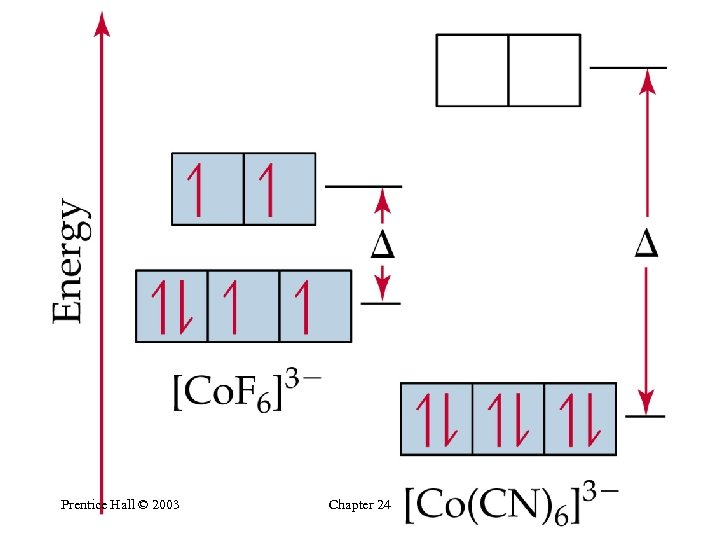Prentice Hall © 2003 Chapter 24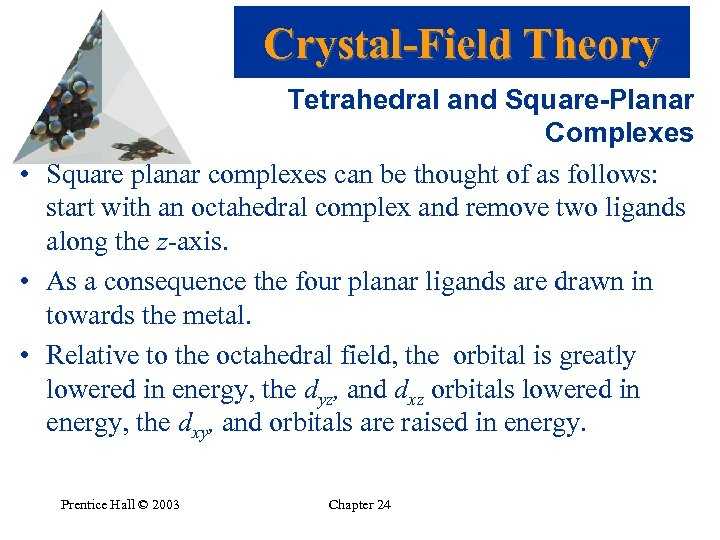Crystal-Field Theory Tetrahedral and Square-Planar Complexes • Square planar complexes can be thought of as follows: start with an octahedral complex and remove two ligands along the z-axis. • As a consequence the four planar ligands are drawn in towards the metal. • Relative to the octahedral field, the orbital is greatly lowered in energy, the dyz, and dxz orbitals lowered in energy, the dxy, and orbitals are raised in energy. Prentice Hall © 2003 Chapter 24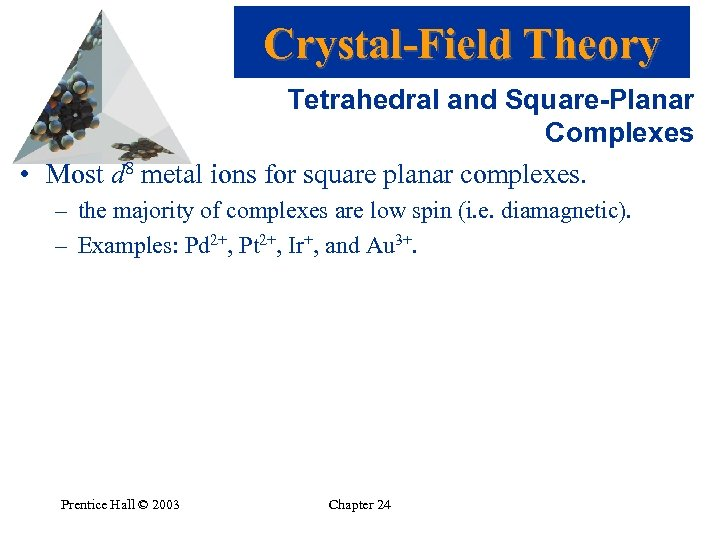Crystal-Field Theory Tetrahedral and Square-Planar Complexes • Most d 8 metal ions for square planar complexes. – the majority of complexes are low spin (i. e. diamagnetic). – Examples: Pd 2+, Pt 2+, Ir+, and Au 3+. Prentice Hall © 2003 Chapter 24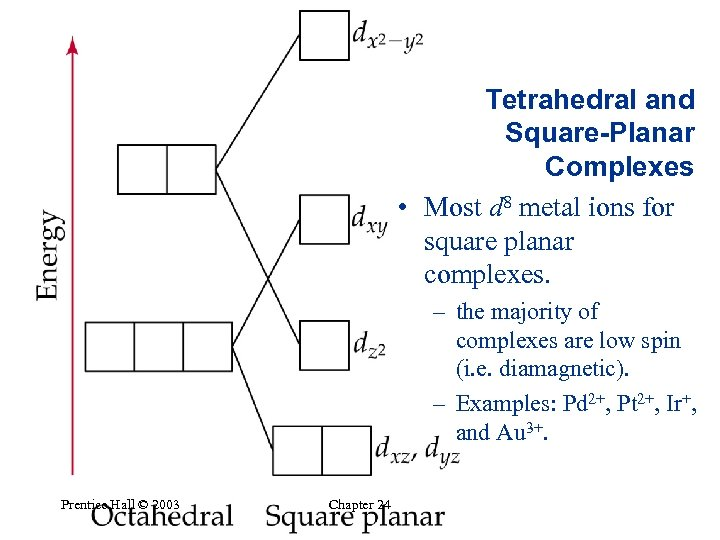Tetrahedral and Square-Planar Complexes • Most d 8 metal ions for square planar complexes. – the majority of complexes are low spin (i. e. diamagnetic). – Examples: Pd 2+, Pt 2+, Ir+, and Au 3+. Prentice Hall © 2003 Chapter 24